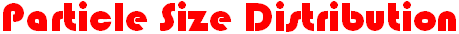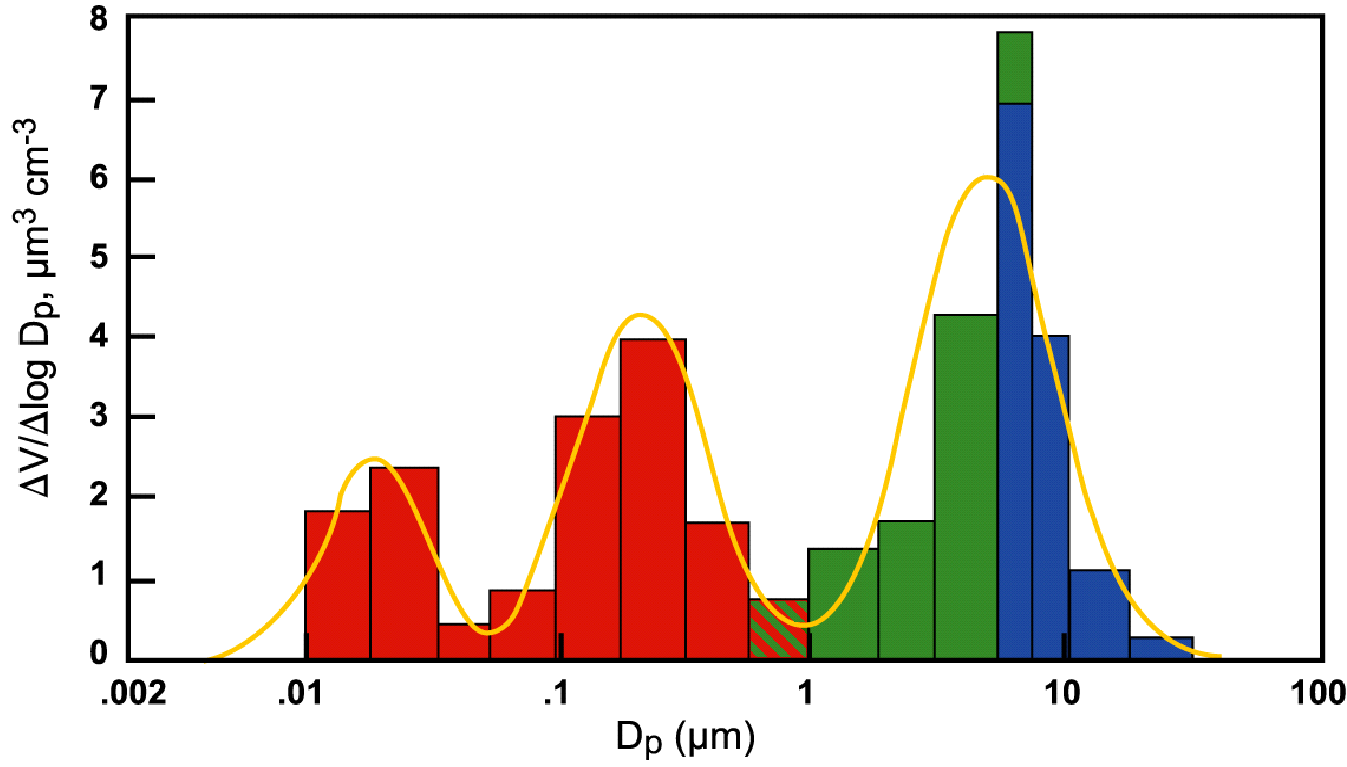The FPM solves for the spatial and temporal evolution of the particle size distribution, which is represented as a multimodal distribution, where each mode represents a distinct population of particles originating from a distinct source. For example, the figure below shows a trimodal particle size distribution measured near a road. The smallest mode represents combustion particles emitted from vehicles, the middle mode represents background particles blowing over the road, and the largest mode represents dirt and tire particles. The FPM uses a single lognormal function to represent the size distribution within each mode. Lognormal distribution functions represent complex size distributions with a minimum of FLUENT scalars, thus permitting computationally efficient simulations. The output of the FPM is the size distribution parameters (e.g., mean size and standard deviation) at each point of interest in the simulation domain.